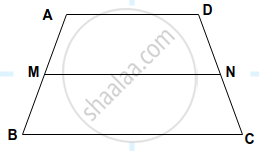# Prove that the median of a trapezium is parallel to the parallel sides of the trapezium and its length is half of the sum of the lengths of the parallel sides. - Mathematics and Statistics

Sum

Prove that the median of a trapezium is parallel to the parallel sides of the trapezium and its length is half of the sum of the lengths of the parallel sides.

#### SolutionLet bar"a", bar"b", bar"c"  "and"  bar"d" be respectively the position vectors of the vertices A, B, C and D of the trapezium ABCD, with side AD || side BC.

Then the vectors bar"AD" and bar"BC" are parallel.

∴ there exists a scalar k,

such that bar"AD" = "k".bar"BC"

∴ bar"AD" + bar"BC" = "k".bar"BC" + bar"BC"

= ("k" + 1)bar"BC"     ....(1)

Let bar"m" and bar"n" be the position vectors of the midpoints M and N of the non-parallel sides AB and DC respectively. Then seg MN is the median of the trapezium.

By the midpoint formula,

bar"m" = (bar"a" + bar"b")/2 and bar"n" = (bar"d" + bar"c")/2

∴ bar"MN" = bar"n" - bar"m"

= ((bar"d" + bar"c")/2) - ((bar"a" + bar"b")/2)

= 1/2 (bar"d" + bar"c" - bar"a" - bar"b")

= 1/2 [(bar"d" - bar"a") + (bar"c" - bar"b")]

= (bar"AD" + bar"BC")/2     .....(2)

= (("k" + 1)bar"BC")/2    ....[By (1)]

Thus bar "MN" is a scalar multiple of bar "BC"

∴ bar "MN" and bar "BC" are parallel vectors

∴ bar "MN" || bar "BC" where bar "BC" || bar "AD"

∴ the median MN is parallel to the parallel sides AD and BC of the trapezium.

Now bar "AD" and bar "BC" are collinear

∴ |bar "AD" + bar "BC"| = |bar "AD"| + |bar "BC"| = "AD" + "BC"

∴ from (2), we have

Now bar "MN" = (bar "AD" + bar "BC")/2

∴ MN = 1/2("AD" + "BC")

Concept: Section Formula
Is there an error in this question or solution?